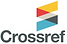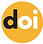top of page## ISSN 2621-5799Published: 20 September 2021

# Examination of Semantic Structures Used by Teacher Candidates to Transform Algebraic Expressions into Verbal Problems

### Ayten Pinar Bal

The University of Cukurova, Turkey10.31014/aior.1993.04.03.355

Pages: 484-494

Keywords: Semantic Structures, Algebraic Expressions, Verbal Problems, Problem Structure, Teacher Candidates

Abstract

The aim of this study is to examine the semantic structures used by mathematics teacher candidates to transform algebraic expressions into verbal problems. The research is a descriptive study in the survey model, which is one of the quantitative research types. The study group of the research consists of 165 teacher candidates studying in the primary school mathematics teaching department of a state university in the south of Turkey in the 2019-2020 academic years. 73.2% of the teacher candidates in the study group are female and 26.8% are male. Criterion sampling method, one of the purposeful sampling methods, was used in the selection of teacher candidates in the study group. While the Algebraic Expression Questionnaire Form was used as the data collection tool, the evaluation rubric of verbal problems was used in the analysis of the data. As a result of the research, it has been revealed that pre-service teachers are more successful in transforming algebraic expressions into verbal problems, but they have problems in creating problems with algebraic expressions that make up systems of equations. Again in the study, it was concluded that pre-service teachers used addition and subtraction problems more than multiplication and division problems. On the other hand, when the problems in the type of addition and subtraction are examined in the study, in the type of combining and separating; It has been concluded that the category of equal groups is mostly used in the problems of multiplication and division.

References

1. Cañadas, M. C., Molina, M. & Río, A. D. (2018). Meanings given to algebraic symbolism in problem-posing. Educ Stud Math, 98:19–37. https://doi.org/10.1007/s10649-017-9797-9

2. Canbazoglu, H. B. & Tarim, K. (2019). Types of verbal problems that primary school teachers’ present to students. Mersin University Journal of the Faculty of Education 15(2), 526-541.

3. Carpenter, T. P. & Moser, J. M. (1982). The development of addition and subtraction problem-solving skills. In T. P. Carpenter, J. M. Moser, & T. Romberg. (Eds.), Addition and subtraction a cognitive perspective. (pp.9-24). Hillsdale, NJ: Erlbaum.

4. Christou, C. & Philippou, G., (1998). The developmental nature of ability to solve one step word problems. Journal for Research in Mathematics Education, 29(4), 436-443.

5. De Corte, E., & Verschaffel, L. (1987). The effect of semantic structure on first graders’ solution strategies of elementary addition and subtraction word problems. Journal for Research in Mathematics Education, 18, 363–381.

6. Dede, Y. (2005). Interpretation of the first-degree equations: A study on freshmen students in education faculty. Cumhuriyet University Social Sciences Journal, 29(2), 197–205.

7. Despina, D. & Loukidou, H. (2014). Addition and subtraction word problems in greek grade a and grade b mathematics textbooks: distribution and children’s understanding. International Journal for Mathematics Teaching and Learning, 8, 340.

8. Duru, A. & Koklu, O. (2011). Middle school students ’ reading comprehension of mathematical texts and algebraic equations. International Journal of Mathematical Education in Science and Technology, 42(4), 37–41. https://doi.org/10.1080/0020739X.2010.550938

9. Fernández-Millána, M. & Molina, M. (2017). Secondary students’ implicit conceptual knowledge of algebraic symbolism. an exploratory study through problem posing. International Electronic Journal of Mathematics Education, 12(3), 799-826.

10. Fraenkel, J. R., Wallen, N. E., & Hyun, H. H. (2012).How to Design and Evaluate Research in Education (8th ed.).New York: McGram-Hill Companies.

11. Haylock, D. ve Cockburn, A. (2008). Understanding mathematics for Young Children. London: SAGE Publications Ltd.

12. Isık, C. & Kar, T. (2012). The analysis of the problems the pre-service teachers experience in posing problems about equations. Australian Journal of Teacher Education, 37(9), 93-113.Available at: http://ro.ecu.edu.au/ajte/vol37/iss9/6

13. Kar, T. (2015). Analysis of problems posed by sixth-grade middle school students for the addition of fractions in terms of semantic structures. International Journal of Mathematical Education in Science and Technology, 46(6), 879-894.

14. Kar, T., Ocal, T., Ocal, M. F. & Demirci, O. (2021). Problem posing with third-grade children: Examining the complexity of problems. International Journal of Contemporary Educational Research, 8(1), 54-71. DOI: https://doi.org/10.33200/ijcer.820714.

15. Katz, J. V. (2007). Stages in the history of algebra with implications for teaching. Educational Studies in Mathematics, 66(1), 185-201

16. Kılıc, C. (2013). Determining performance of elementary students related to problem posing activities requiring four arithmetical operations with natural numbers. Dicle Universitesi Ziya Gokalp Egitim Fakultesi Dergisi, 20, 256-274.

17. Kılıc, C. (2013). Turkish primary school teachers’ opinions about problem using applications: students, the mathematics curriculum and mathematics textbooks, Australian Journal of TeacherEducation, 38(5), http://dx.doi.org/10.14221/ajte.2013v38n5.10

18. Kieran C (1992) The learning and teaching of school algebra. In: Grouws DA (ed) Handbook of research on mathematics teaching and learning. Macmillan, New York, pp 390–419

19. Kieran C (2007) Learning and teaching algebra at the middle school through college levels: Building meaning for symbols and their manipulation. In: F.K Lester (Ed.) Second handbook of research on mathematics teaching and learning(pp 707–762). Charlotte, NC: Information Age Publishing

20. Marshall, S. P. (1995). Schemas in problem solving. New York, NY: Cambridge University Press.

21. Miles, M. B. & Huberman, M.A. (1994). An Expanded Sourcebook Qualitative Data Analysis.London: Sage Publication.

22. National Council of Teachers of Mathematics (NCTM). (2000). Principles and standards for school mathematics.Reston, VA: NCTM

23. Olkun, S. & Toluk, Z. (2002). Textbooks, word problems and student success on addition and subtraction. International Journal for Mathematics Teaching and Learning., 1-8.https://www.cimt.org.uk/journal/olkuntoluk.pdf

24. Parmjit, S. & Teoh, S. H. (2010). An analysis of addition and subtraction word problems in mathematics textbooks used in Malaysian primary school classrooms. Brunei lnt.J. of Sci. & Math. Edu., 2(1), 68- 85.

25. Patton, M. Q. (2014). Qualitative Research & Evaluation Methods. London: Sage Publications Inc.

26. Pilten, P. (2010). Evaluation of operation techniques used for the solution of problems with different semantic structure. e-Journal of New World Sciences Academy Education Sciences, 5(3)